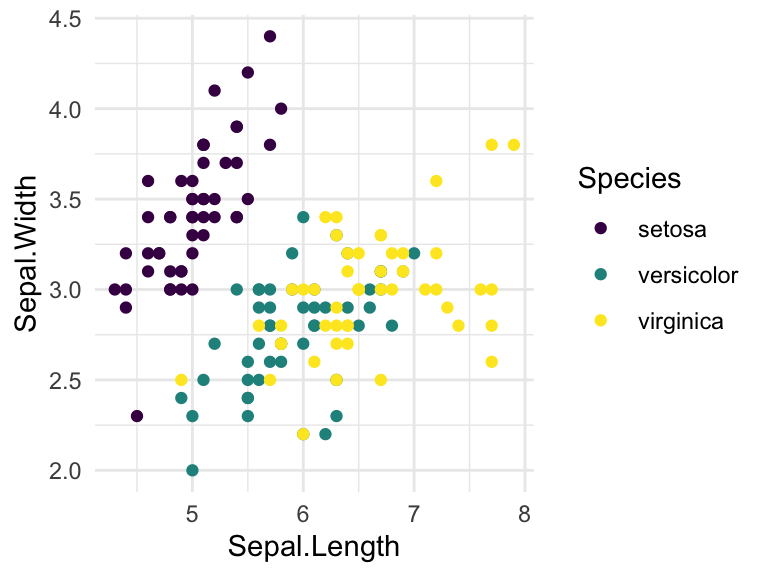# How to Install GGPLOT2 in R#### Related Book

GGPlot2 Essentials for Great Data Visualization in R

## Installing ggplot2

The ggplot2 package can be easily installed using the R function install.packages().

You need to type the following R code in the console:

install.packages("ggplot2")

The above code will automatically download the ggplot2 package, from the CRAN (Comprehensive R Archive Network) repository, and install it.

## Using ggplot2

After installing the package, you can load it using the R function library().

# Load ggplot2
library("ggplot2")

# Create a scatter plot
ggplot(iris, aes(x = Sepal.Length, y = Sepal.Width)) +
geom_point(aes(color = Species)) +
scale_color_viridis_d() +
theme_minimal()